# Divisor count function

(diff) ← Older revision | Latest revision (diff) | Newer revision → (diff)
This article defines an arithmetic function or number-theoretic function: a function from the natural numbers to a ring (usually, the ring of integers, rational numbers, real numbers, or complex numbers).
View a complete list of arithmetic functions

## Definition

Letbe a natural number. The divisor count function of, denoted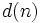, or, is defined as the number of positive divisors of. In other words:.

### Formula in terms of prime factorization

Suppose we have:.

Then: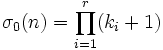.

## Behavior

### Lower bound

The divisor count function oftakes its lowest value (other than) at primes.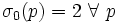.

In particular: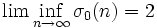.

### Upper bound

Fill this in later

## Relation with other arithmetic functions

### Family of divisor power sum functions

For any real number (typically, integer), the divisor power sum function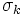is the sum ofpowers of all the positive divisors of. The divisor count function is the special case. The case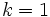is the divisor sum function, often just denoted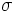, while the case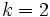is the divisor square sum function.

## Relation with properties of numbers

• Prime number is a natural numberfor which.
• Perfect square is a natural numberfor whichis odd.
• Refactorable number is a natural numberfor whichdivides.

## Properties

### Multiplicativity

This arithmetic function is a multiplicative function: the product of this function for two natural numbers that are relatively prime is the value of the function at the product.
View a complete list of multiplicative functions

### Complete multiplicativity

NO: This arithmetic function is not a completely multiplicative function: in other words, the product of the values of the function at two natural numbers need not equal the value at the product.

For natural numbersand, it is not necessary that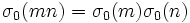. In fact, the equality holds if and only ifandare relatively prime.

### Preservation of divisibility

NO: This arithmetic function is not a divisibility-preserving function: it does not preserve divisibility.

Ifdivides, it is not necessary thatshould divide.

## Generalization to other rings

This generalizes the notion of divisor count function from the case of the ring of integersto more general classes of rings.

### Unique factorization domains

Supposeis a unique factorization domain. The divisor count function is a function defined on the nonzero elements of, and is defined as the number of associate classes of divisors of that element. (Note that this does not count the actual number of divisors, but only the number of divisors up to multiplication by units -- this has the same effect as counting only the positive divisors does in the case of integers). Further, the same formula in terms of factorization holds. If we have: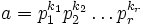.

Then: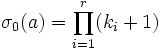.

## Algebraic significance

• Number of subgroups of the cyclic group: For any natural number,equals the number of subgroups of the cyclic group of orderunder the action of the automorphism group.
• Number of automorphism classes of elements in the cyclic group: For any natural number,equals the number of equivalence classes of elements in the cyclic group of orderunder the action of the automorphism group. In fact, two elements are in the same automorphism class if and only if they generate the same subgroup. The sizes of these equivalence classes arefor the divisorsof, and this is a combinatorial proof of the fact that.
• Number of associate classes of elements in the ring of integers modulo: For any natural number,equals the number of equivalence classes of elements in the ring of integers modulounder the relation of being associate elements. In fact, the equivalence classes of associate elements are precisely the same as the equivalence classes under the action of automorphisms of the additive group of the ring. Thus, their sizes are, for the divisorsof.
• Number of irreducible factors of the polynomialover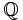: This polynomial is a product of irreducible factors called cyclotomic polynomialsfor the divisorsof, wherehas as its roots the primitiveroots of unity. The degree ofis.